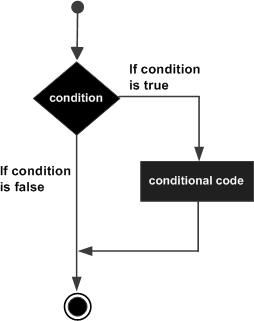# if statement in java

An if statement consists of a Boolean expression followed by one or more statements.

## Syntax

Following is the syntax of an if statement −

```if(Boolean_expression) {
// Statements will execute if the Boolean expression is true
}
```

If the Boolean expression evaluates to true then the block of code inside the if statement will be executed. If not, the first set of code after the end of the if statement (after the closing curly brace) will be executed.

## Flow Diagram## Example

```public class Test {

public static void main(String args[]) {
int x = 10;

if( x < 20 ) {
System.out.print("This is if statement");
}
}
}
```

This will produce the following result −

## Output

```This is if statement.
```
java_decision_making.htm